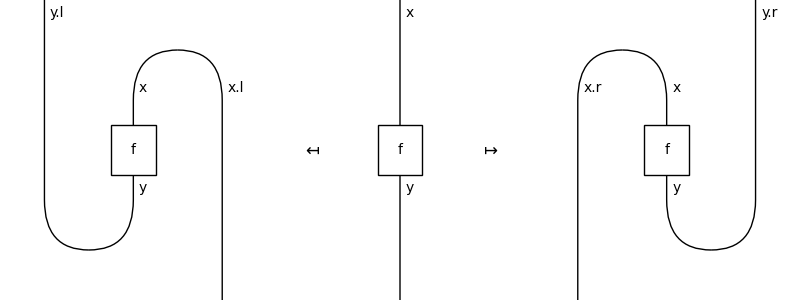# Diagram#

class discopy.interaction.Diagram(inside, dom, cod)[source]#

Bases: `Composable`[`Ty`], `Whiskerable`, `NamedGeneric['natural']`

An integer diagram from `x` to `y` is a `natural` diagram from `x.positive @ y.negative` to `x.negative @ y.positive`.

Parameters
• inside (natural) – The natural diagram inside.

• dom (Ty) – The domain of the diagram, i.e. its input.

• cod (Ty) – The codomain of the diagram, i.e. its output.

Note

By default we take `natural = ribbon.Diagram` but this can be any class with the methods for a balanced traced category. For example, the category of boolean matrices with the direct sum has a trace given by reflexive transitive closure. We can use it to check the snake equations:

```>>> from discopy.matrix import Matrix
>>> T, D = Ty[int], Diagram[Matrix[bool]]
>>> assert D.id(T(2, 2)).transpose()\
...     == D.id(T(2, 2))\
...     == D.id(T(2, 2)).transpose(left=True)
```
natural#

alias of `Diagram`

then(other)[source]#

The composition of two integer diagrams.

Parameters

other (Diagram) – The other diagram with which to compose.

Example

```>>> from discopy.ribbon import Ty as T, Diagram as D, Box as B
>>> u, v, w, x, y, z = map(Ty[T], "uvwxyz")
>>> f = Diagram[D](B('f', T('x', 'v'), T('y', 'u')), x @ -u, y @ -v)
>>> g = Diagram[D](B('g', T('y', 'w'), T('z', 'v')), y @ -v, z @ -w)
>>> (f >> g).draw(path='docs/_static/int/composition.png')
```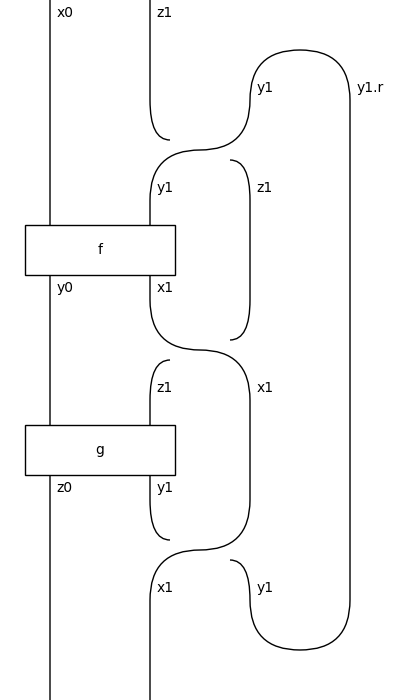classmethod id(dom=None)[source]#

The identity on an integer type.

Parameters

dom (Optional[Ty]) – The integer type on which to take the identity.

Return type

Diagram

Example

```>>> from discopy.ribbon import Ty as T, Diagram as D, Box as B
>>> x, y, u, v = map(Ty[T], "xyuv")
>>> f = Diagram[D](B('f', T('x', 'v'), T('y', 'u')), x @ -u, y @ -v)
>>> (Diagram[D].id(x @ -u) >> f).draw(path='docs/_static/int/idl.png')
```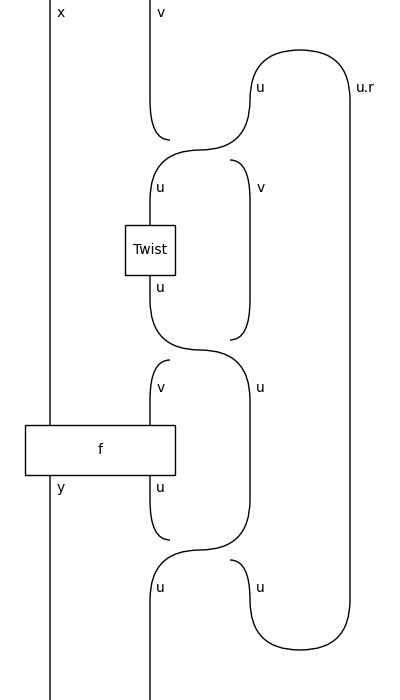```>>> (f >> Diagram[D].id(y @ -v)).draw(path='docs/_static/int/idr.png')
```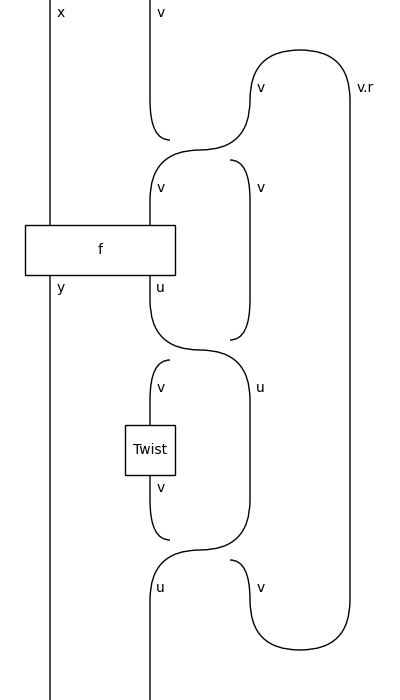tensor(other)[source]#

The tensor of two integer diagrams.

Parameters

other – The other diagram to tensor.

Example

```>>> from discopy.ribbon import Ty as T, Diagram as D, Box as B
>>> x, y, u, v = map(Ty[T], "xyuv")
>>> x_, y_, u_, v_ = map(lambda x: Ty[T](x + '_'), "xyuv")
>>> f = Diagram[D](B('f', T('x', 'v'), T('y', 'u')), x @ -u, y @ -v)
>>> f_ = Diagram[D](
...     B('f_', T('x_', 'v_'), T('y_', 'u_')), x_ @ -u_, y_ @ -v_)
>>> (f @ f_).draw(path='docs/_static/int/tensor.png')
```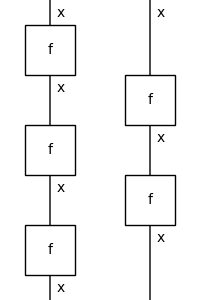classmethod braid(left, right)[source]#

The braid of integer diagrams is given by the following diagram:

```>>> from discopy.ribbon import Ty as T, Diagram as D, Box as B
>>> x, u, y, v = map(Ty[T], "xuyv")
>>> Diagram.braid(x @ -u, y @ -v).draw(
...     path="docs/_static/int/braid.png")
```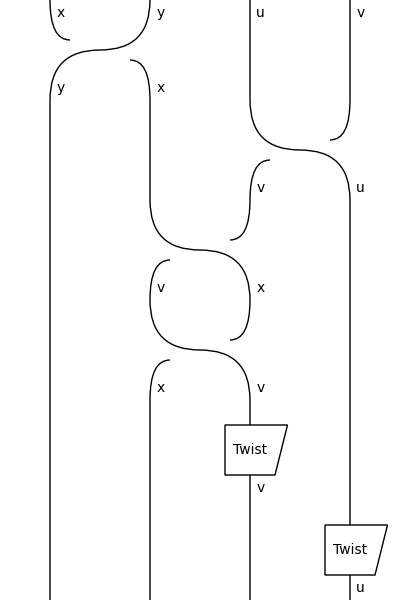Parameters
• left (Ty) – The left input of the braid.

• right (Ty) – The right input of the braid.

Return type

Diagram

classmethod cups(left, right)[source]#

The integer cups are given by natural identities.

Parameters
• left (Ty) – The left-hand side of the cups.

• right (Ty) – The right-hand side of the cups.

Return type

Diagram

Example

This is what the snake equations look like:

```>>> from discopy.drawing import Equation
>>> x = Ty('x')
>>> Equation(
...     Diagram.caps(x, -x) @ x >> x @ Diagram.cups(-x, x),
...     Diagram.id(x),
...     x @ Diagram.caps(-x, x) >> Diagram.cups(x, -x) @ x).draw(
...         path="docs/_static/int/int-snake-equations.png")
```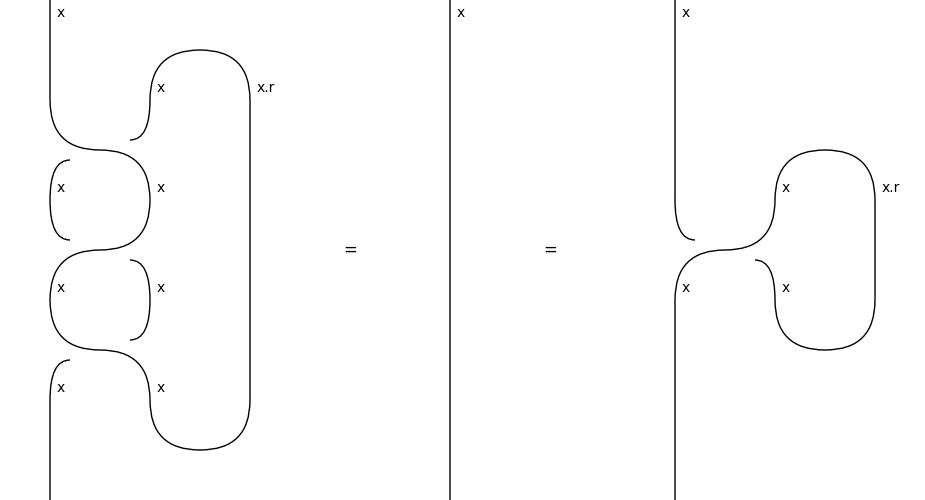classmethod caps(left, right)[source]#

The integer caps are given by natural identities.

Parameters
• left (Ty) – The left-hand side of the caps.

• right (Ty) – The right-hand side of the caps.

Return type

Diagram

dagger()[source]#

The dagger of an integer diagram is given by the dagger of its inside.

```>>> from discopy.ribbon import Ty as T, Diagram as D, Box as B
>>> x, y, u, v = map(Ty[T], "xyuv")
>>> f = Diagram[D](B('f', T('x', 'v'), T('y', 'u')), x @ -u, y @ -v)
>>> from discopy.drawing import Equation
>>> Equation(f, f[::-1], symbol="\$\\mapsto\$").draw(
...     path="docs/_static/int/dagger.png")
```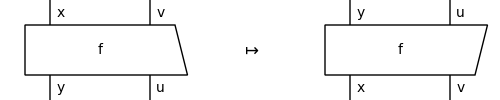draw(**params)[source]#

The drawing of an integer diagram is the drawing of its inside.

simplify()[source]#

Simplify by going back and forth to `Hypergraph`.

Example

```>>> x = Ty[frobenius.Ty]('x')
>>> D = Diagram[frobenius.Diagram]
>>> left_snake = D.id(-x).transpose(left=True)
>>> right_snake = D.id(-x).transpose(left=False)
>>> assert left_snake.simplify() == D.id(x) == right_snake.simplify()
```
```>>> from discopy.drawing import Equation
>>> Equation(left_snake, Equation(
...     D.id(x), right_snake, symbol="\$\\leftarrow\$"),
...         symbol="\$\\rightarrow\$").draw(
...             path="docs/_static/int/simplify.png")
```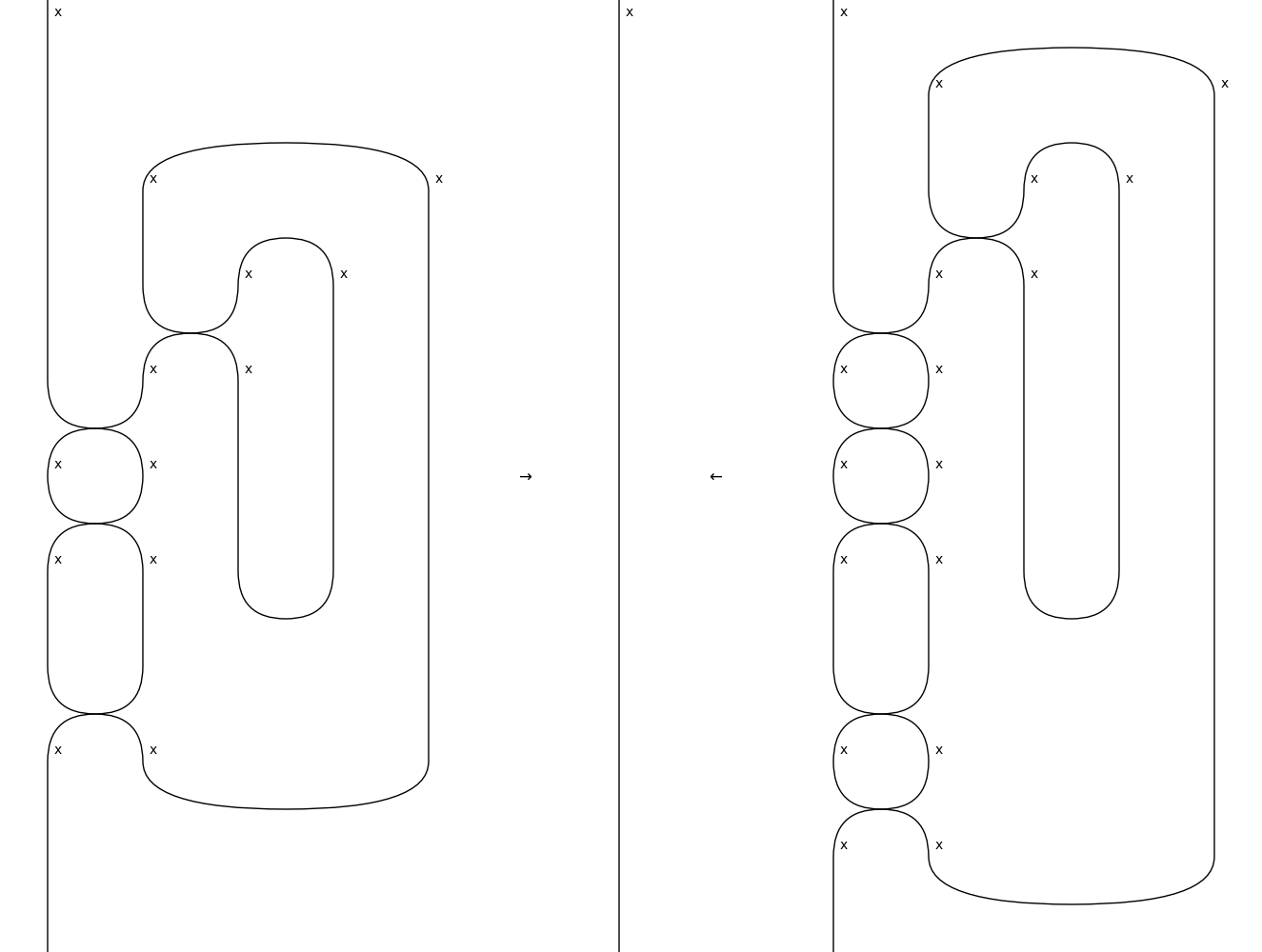naturality(i, left=True, down=True, braid=None)[source]#

Slide a box through a braid.

Parameters
• i (int) – The index of the box to slide.

• left – Whether to slide left or right.

• down – Whether to slide down or up.

• braid – The braiding method to be used.

Return type

Diagram

Examples

```>>> x, y, z = map(Ty, "xyz")
>>> f = Box('f', x, y)
>>> top_left = f @ z >> Braid(y, z)
>>> top_right = z @ f >> Braid(z, y)
>>> bot_left = Braid(z, x) >> f @ z
>>> bot_right = Braid(x, z) >> z @ f
>>> assert top_right.naturality(0) == bot_left
>>> assert top_left.naturality(0, left=False) == bot_right
>>> assert bot_right.naturality(1, down=False) == top_left
>>> assert bot_left.naturality(1, left=False, down=False) == top_right
```
trace(n=1, left=False)[source]#

Feed `n` outputs back into inputs.

Parameters
• n – The number of output wires to feedback into inputs.

• left – Whether to trace the wires on the left or right.

Example

```>>> from discopy.drawing import Equation as Eq
>>> x = Ty('x')
>>> f = Box('f', x @ x, x @ x)
>>> LHS, RHS = f.trace(left=True), f.trace(left=False)
>>> Eq(Eq(LHS, f, symbol="\$\\mapsfrom\$"),
...     RHS, symbol="\$\\mapsto\$").draw(
...         path="docs/_static/traced/trace.png")
```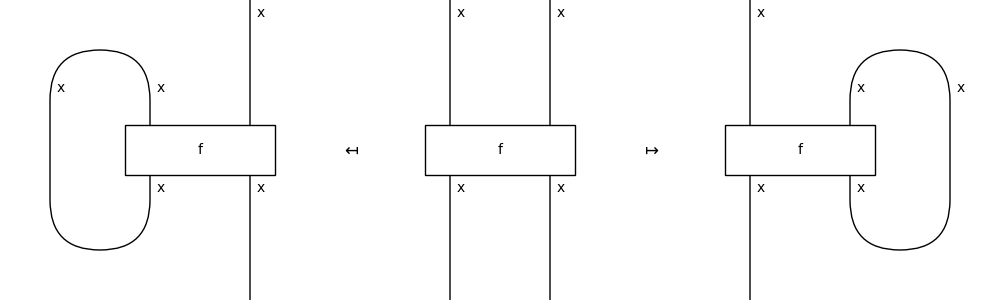classmethod trace_factory(diagram, left=False)[source]#

The trace of a pivotal diagram is its pre- and post-composition with cups and caps to form a feedback loop.

Parameters
• diagram (Diagram) – The diagram to trace.

• left – Whether to trace on the left or right.

transpose(left=False)[source]#

The transpose of a diagram, i.e. its composition with cups and caps.

Parameters

left – Whether to transpose left or right.

Example

```>>> from discopy.drawing import Equation
>>> x, y = map(Ty, "xy")
>>> f = Box('f', x, y)
>>> LHS = Equation(f.transpose(left=True), f, symbol="\$\\mapsfrom\$")
>>> RHS = Equation(LHS, f.transpose(), symbol="\$\\mapsto\$")
>>> RHS.draw(figsize=(8, 3), path="docs/_static/rigid/transpose.png")
```